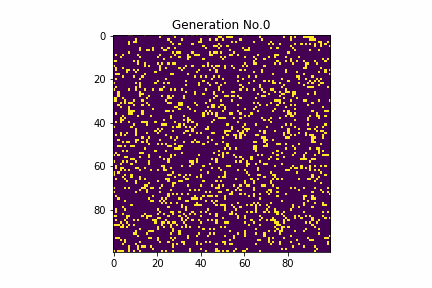# Save the result of the life game as a gif with python

It's a story of \$ n \$ decoction, but in this article I will give a brief explanation of Life Game and try to simulate it with python. Then save the state of each era as a figure and combine it as a gif to make a simple animation.

## What is a life game?

Conway's Game of Life is simply a simulation of the survival of living things. The plane is divided by a grid, and each square holds two states, with or without living things. The state changes with the following rules for the distribution of living things every unit time.

・ In the surrounding 8 squares excluding yourself in a certain square If there is only one organism → death (depopulation) If there are two → as it is (survival) If there are 3 animals → a creature will be born in that square (birth) If 4 or more → Death (overcrowding) 　 Despite these simple rules, it creates a very interesting pattern as a whole. First, let's take a look at the gif output from the program in this article.Not only does it increase or decrease the number of creatures, but it also creates and moves special patterns. It's interesting just looking at it. Even though individual organisms follow simple laws, they move in a complex manner that cannot be reduced to individual simple movements as a whole ... Such a system is called a complex system.

## program

### Module import and result output destination

#### `1.py`

``````
#Modules
from random import randint
import matplotlib.pyplot as plt
import numpy as np
import os
from PIL import Image

#Output destination of simulation results
di = "./"
pics = di + "pictures/"
gif = di + "simulation_results/"

#Folder creation
try:
os.mkdir(pics)
os.mkdir(gif)
except:
pass
``````

### Make a diagram of the result and save it

#### `2.py`

``````
#Make a diagram of the result and save it
#The argument is a value indicating the state of the plane in the t era and the generation it is.
def make_fig(world,t):
picsfname = pics + str(t) + ".png "
pictitle = "Generation No." + str(t)
plt.figure()
plt.title(pictitle)
#Plot on a two-dimensional plane
plt.imshow(world)
plt.savefig(picsfname)
#Do not display the figure
plt.close()
``````

### Explore the surrounding squares

#### `3.py`

``````
def serch(world,x,y):
#counter
c = 0

#search
for i in range(x-1,x+2):
for j in range(y-1,y+2):
c += world[i][j]
c -= world[x][y]

#Survive if 3 animals are in the surroundings, remain as it is if 2 animals, die otherwise
if c == 3:
return 1
elif c == 2:
return  world[x][y]
else:
return 0
``````

### Initial field generation

#### `4.py`

``````
#The initial position of the organism is determined by a random number
for i in range(d):
tmp = []
for j in range(d):
#The larger the number of divisions, the fewer early creatures
if randint(0,100)%7 == 0:
tmp.append(1)
else:
tmp.append(0)
world.append(tmp)
``````

### Receiving parameters

#### `5.py`

``````
#Parameter input
#Many frames and number of repeating generations
d = int(input("Frame size"))
n = int(input("Generations?"))
world = []
``````

### Main part

#### `4.py`

``````
#Record the initial state
make_fig(world,0)

#Main loop
for t in range(1,n):
#Generate fields from outputting fields in the following states
newworld = []
for i in range(d):
tmp = []
for j in range(d):
tmp.append(0)
newworld.append(tmp)
#Explore each square in the field
for i in range(1,d-1):
for j in range(1,d-1):
newworld[i][j] = serch(world,i,j)
#Transfer state to new field
for i in range(d):
for j in range(d):
world[i][j] = newworld[i][j]
#Finally save the state
make_fig(world,t)
``````

### Generate gif animation by grouping images

#### `6.py`

``````
#./Connect the figures saved in pictures to make a gif
frames = []
#./simulation_results/Output to
giffname = gif + "simulation.gif"

for i in range(n):
picname = pics + str(i) + ".png "
new_frame = Image.open(picname)
frames.append(new_frame)

#gif
frames.save(giffname,
format='GIF',
append_images=frames[1:],
save_all=True,
duration=400,
loop=0)

#Reference: https://blog.fantom.co.jp/2019/12/28/make-gif-animation-from-multiple-images/
``````

### Overall

#### `7.py`

``````
#Modules
from random import randint
import matplotlib.pyplot as plt
import numpy as np
import os
from PIL import Image

#Output destination of simulation results
di = "./"
pics = di + "pictures/"
gif = di + "simulation_results/"

#Folder creation
try:
os.mkdir(pics)
os.mkdir(gif)
except:
pass

#Make a diagram of the result and save it
def make_fig(world,t):
picsfname = pics + str(t) + ".png "
pictitle = "Generation No." + str(t)
plt.figure()
plt.title(pictitle)
plt.imshow(world)
plt.savefig(picsfname)
plt.close()

#Search for 8 squares around you and check for the presence of living things
def serch(world,x,y):
#counter
c = 0

#search
for i in range(x-1,x+2):
for j in range(y-1,y+2):
c += world[i][j]
c -= world[x][y]

#Survive if 3 animals are in the surroundings, remain as it is if 2 animals, die otherwise
if c == 3:
return 1
elif c == 2:
return  world[x][y]
else:
return 0

#Parameter input
d = int(input("Frame size"))
n = int(input("Generations?"))
world = []

#Field generation
#The initial position of the organism is determined by a random number
for i in range(d):
tmp = []
for j in range(d):
if randint(0,100)%7 == 0:
tmp.append(1)
else:
tmp.append(0)
world.append(tmp)

#Record the initial state
make_fig(world,0)

#Main loop
for t in range(1,n):
newworld = []
for i in range(d):
tmp = []
for j in range(d):
tmp.append(0)
newworld.append(tmp)

for i in range(1,d-1):
for j in range(1,d-1):
newworld[i][j] = serch(world,i,j)

for i in range(d):
for j in range(d):
world[i][j] = newworld[i][j]
make_fig(world,t)

#./Connect the figures saved in pictures to make a gif
frames = []
giffname = gif + "simulation.gif"

for i in range(n):
picname = pics + str(i) + ".png "
new_frame = Image.open(picname)
frames.append(new_frame)

#gif
frames.save(giffname,
format='GIF',
append_images=frames[1:],
save_all=True,
duration=400,
loop=0)
print("finished")
``````